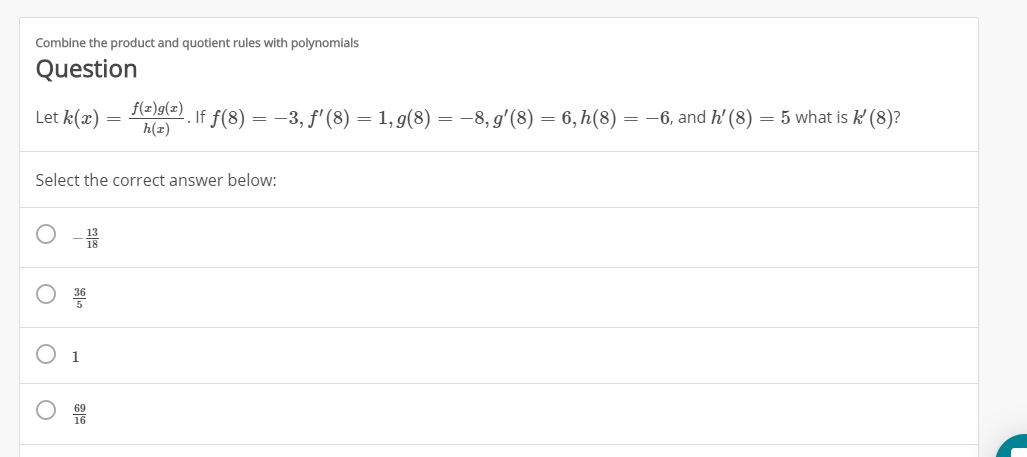# Combine the product and quotient rules with polynomialsQuestionaf f(8)-3, f'(8) = 1, g(8) = -8,g'(8) = 6,h(8) = -6, and h' (8)Let k(ax)5 what is k (8)?h(zSelect the correct answer below1

Question
1 viewshelp_outlineImage TranscriptioncloseCombine the product and quotient rules with polynomials Question a f f(8)-3, f'(8) = 1, g(8) = -8,g'(8) = 6,h(8) = -6, and h' (8) Let k(ax) 5 what is k (8)? h(z Select the correct answer below 1 fullscreen
check_circle

star
star
star
star
star
1 Rating
Step 1

Apply quotient rule on the right side.

Step 2

Apply product rule on [f(x)...help_outlineImage Transcriptionclosek'(x)f(x)g(x)]'h(x)-f(x)g(x)h'(x) h(x)] [f'(x)g(x)+f(x)g'(x)] h(x)- f (x)g(x)h'(x) k'(x)= h(x)]2 f'(x)g(x)h(x)+f(x)g'(x)h(x)- f (x) g(x)h'(x) k'(x)= h(x)] fullscreen

### Want to see the full answer?

See Solution

#### Want to see this answer and more?

Solutions are written by subject experts who are available 24/7. Questions are typically answered within 1 hour.*

See Solution
*Response times may vary by subject and question.
Tagged in

### Other